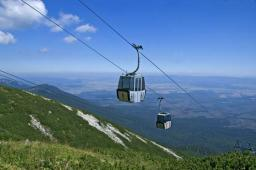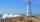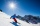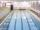# Map - climb

On the map of High Tatras in scale 1:11000 are cable car stations in the Tatranska Lomnica and in the Skalnate Pleso with distance 354.6 mm. Altitude of this stations are 949 m and 1760 m.

What is average angle of climb of this cable car track?

Correct result:

α =  11.75 °

#### Solution:We would be pleased if you find an error in the word problem, spelling mistakes, or inaccuracies and send it to us. Thank you!Tips to related online calculators

#### You need to know the following knowledge to solve this word math problem:

We encourage you to watch this tutorial video on this math problem:

## Next similar math problems:

• Reference angleFind the reference angle of each angle:
• Center traverseIt is true that the middle traverse bisects the triangle?
• How farFrom the top of a lighthouse 145 ft above sea level, the angle of depression of a boat 29°. How far is the boat from the lighthouse?
• CosineThe point (8, 6) is on the terminal side of angle θ. cos θ = ?
• High wallI have a wall 2m high. I need a 15 degree angle (upward) to second wall 4 meters away. How high must the second wall?On a straight stretch of road is marked 12 percent drop. What angle makes the direction of the road with the horizontal plane?
• PyramidPyramid has a base a = 5cm and height in v = 8 cm. a) calculate angle between plane ABV and base plane b) calculate angle between opposite side edges.
• MapleMaple peak is visible from a distance 3 m from the trunk from a height of 1.8 m at angle 62°. Determine the height of the maple.
• Black diamond runTaleah is skiing down a black diamond run. She begins skiing at the top of a ski trail whose elevation is about 8625 feet. The ski run ends toward the base of the mountain at 3800 feet. The horizontal distance between these two points is about 4775 feet.
• Angles of elevationFrom points A and B on level ground, the angles of elevation of the top of a building are 25° and 37° respectively. If |AB| = 57m, calculate, to the nearest meter, the distances of the top of the building from A and B if they are both on the same side of
• TreeHow tall is the tree that observed in the visual angle of 52°? If I stand 5 m from the tree and eyes are two meters above the ground.
• StairwayWhat angle rising stairway if step height in 20 cm and width 26 cm?Average climb of the road is given by ratio 1:15. By what angle road average climb?Circular reflector throws light cone with a vertex angle 49° and is on 33 m height tower. The axis of the light beam has with the axis of the tower angle 30°. What is the maximum length of the illuminated horizontal plane?Calculate slope (rise:run) of the bottom of swimming pool long 30 m. Water depth at beginning of pool is 1.13 m (for children) and depth at end is 1.84 m (for swimmers). Slope express as percentage and as angle in degrees.I have bevel in the ratio 1:6. What is the angle and how do I calculate it?Road has climbing 1:27. How big is a angle corresponds to this climbing?